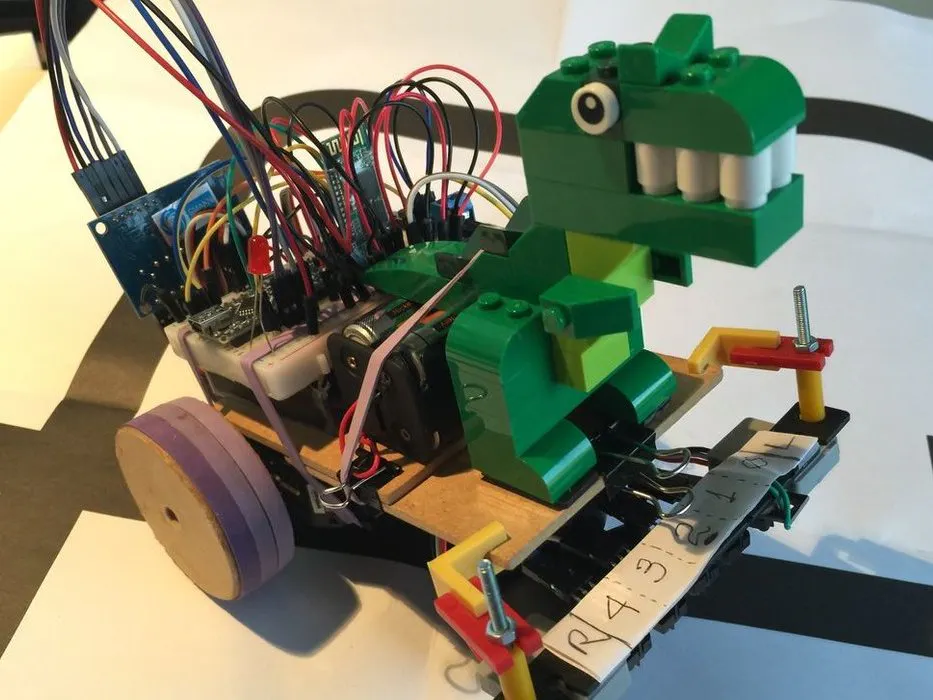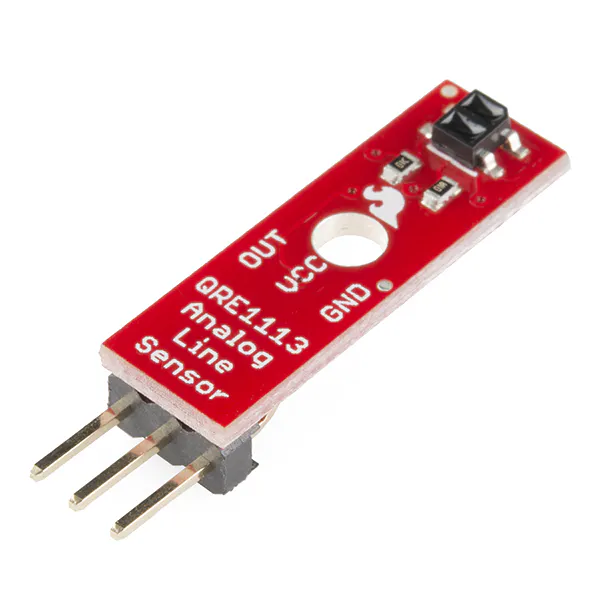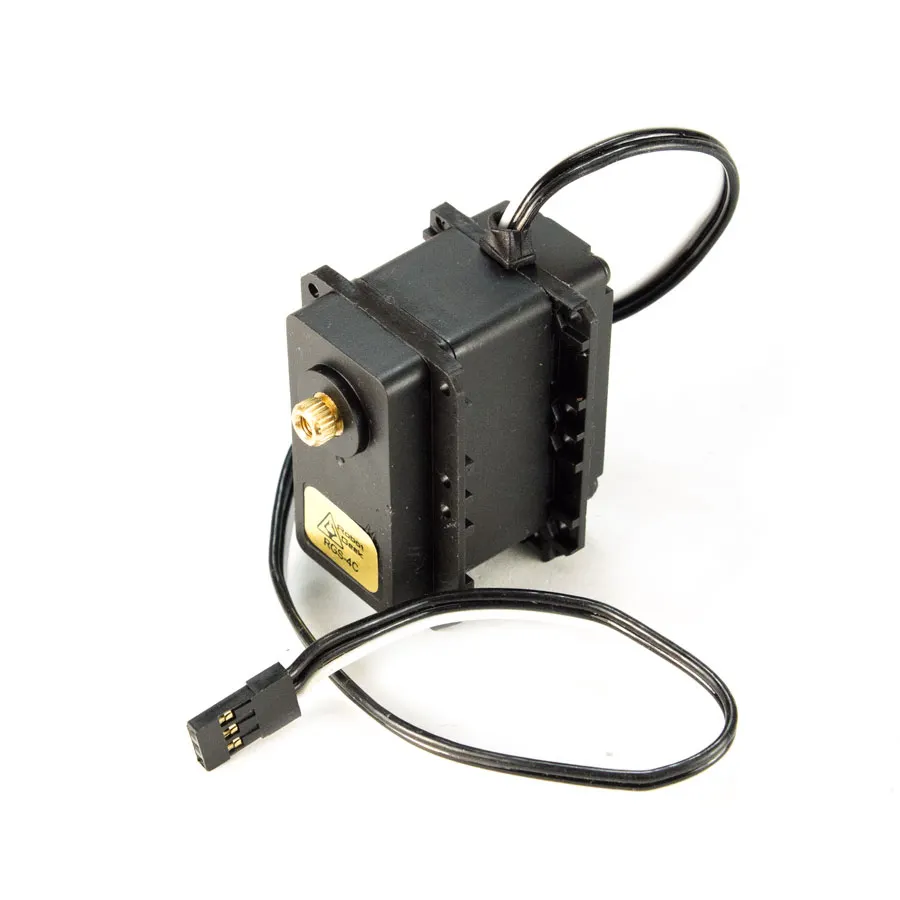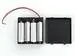# Maze Solver Robot, using Artificial Intelligence

"Rex, the Robot" will try finding how to scape from a "labyrinth" on the shortest and fastest way.

IntermediateFull instructions provided2 days54,291## Things used in this project

### Hardware componentsArduino Nano R3
×1
 HC-06 Bluetooth Module
×1
 TCRT5000 4CH Infrared Line Track Follower Sensor Module
×1SparkFun RedBot Sensor - Line Follower
×1
 ZX03 (based on TCRT5000) Reflective Infrared Sensors (Analog output)
×2Android device
×1RobotGeek Continuous Rotation Servo
×24xAA battery holder
×2

### Software apps and online servicesArduino IDEMIT App Inventor 2

## Code

### Code snippet #1

Plain text
```const int lineFollowSensor0 = 12; //Using Digital input
const int lineFollowSensor1 = 18; //Using Analog Pin A4 as Digital input
const int lineFollowSensor2 = 17; //Using Analog Pin A3 as Digital input
const int lineFollowSensor3 = 16; //Using Analog Pin A2 as Digital input
const int lineFollowSensor4 = 19; //Using Analog Pin A5 as Digital input
const int farRightSensorPin = 0;  //Analog Pin A0
const int farLeftSensorPin = 1;   //Analog Pin A1
```

### Code snippet #4

Plain text
```LFSensor = digitalRead(lineFollowSensor0);
```

### Code snippet #5

Plain text
```void testSensorLogic(void)
{
Serial.print (farLeftSensor);
Serial.print (" <== LEFT RIGH==> ");
Serial.print (farRightSensor);
Serial.print (" mode: ");
Serial.print (mode);
Serial.print (" error:");
Serial.println (error);
}
```

### Code snippet #6

Plain text
```void loop()
{
switch (mode)
{
case NO_LINE:
motorStop();
goAndTurn (LEFT, 180);
break;
case CONT_LINE:
runExtraInch();
if (mode == CONT_LINE) mazeEnd();
else goAndTurn (LEFT, 90); // or it is a "T" or "Cross"). In both cases, goes to LEFT
break;
case RIGHT_TURN:
runExtraInch();
if (mode == NO_LINE) goAndTurn (RIGHT, 90);
break;
case LEFT_TURN:
goAndTurn (LEFT, 90);
break;
case FOLLOWING_LINE:
followingLine();
break;
}
}
```

### Code snippet #7

Plain text
```void runExtraInch(void)
{
motorPIDcontrol();
delay(extraInch);
motorStop();
}
```

### Code snippet #8

Plain text
```void goAndTurn(int direction, int degrees)
{
motorPIDcontrol();
motorTurn(direction, degrees);
}
```

### Code snippet #12

Plain text
```void mazeSolve(void)
{
while (!status)
{
switch (mode)
{
case NO_LINE:
motorStop();
goAndTurn (LEFT, 180);
recIntersection('B');
break;

case CONT_LINE:
runExtraInch();
if (mode != CONT_LINE) {goAndTurn (LEFT, 90); recIntersection('L');} // or it is a "T" or "Cross"). In both cases, goes to LEFT
else mazeEnd();
break;

case RIGHT_TURN:
runExtraInch();
if (mode == NO_LINE) {goAndTurn (RIGHT, 90); recIntersection('R');}
else recIntersection('S');
break;

case LEFT_TURN:
goAndTurn (LEFT, 90);
recIntersection('L');
break;

case FOLLOWING_LINE:
followingLine();
break;

}
}
}
```

### Code snippet #13

Plain text
```void recIntersection(char direction)
{
path[pathLength] = direction; // Store the intersection in the path variable.
pathLength ++;
simplifyPath(); // Simplify the learned path.
}
```

### Code snippet #14

Plain text
```void simplifyPath()
{
// only simplify the path if the second-to-last turn was a 'B'
if(pathLength < 3 || path[pathLength-2] != 'B')
return;

int totalAngle = 0;
int i;
for(i=1;i<=3;i++)
{
switch(path[pathLength-i])
{
case 'R':
totalAngle += 90;
break;
case 'L':
totalAngle += 270;
break;
case 'B':
totalAngle += 180;
break;
}
}

// Get the angle as a number between 0 and 360 degrees.
totalAngle = totalAngle % 360;

// Replace all of those turns with a single one.
switch(totalAngle)
{
case 0:
path[pathLength - 3] = 'S';
break;
case 90:
path[pathLength - 3] = 'R';
break;
case 180:
path[pathLength - 3] = 'B';
break;
case 270:
path[pathLength - 3] = 'L';
break;
}

// The path is now two steps shorter.
pathLength -= 2;

}
```

### Code snippet #15

Plain text
```void loop()
{

mazeSolve(); // First pass to solve the maze
pathIndex = 0;
status = 0;

mazeOptimization(); // Second Pass: run the maze as fast as possible
mode = STOPPED;
status = 0; // 1st pass
pathIndex = 0;
pathLength = 0;
}
```

### Code snippet #16

Plain text
```void mazeOptimization (void)
{
while (!status)
{
switch (mode)
{
case FOLLOWING_LINE:
followingLine();
break;
case CONT_LINE:
if (pathIndex >= pathLength) mazeEnd ();
else {mazeTurn (path[pathIndex]); pathIndex++;}
break;
case LEFT_TURN:
if (pathIndex >= pathLength) mazeEnd ();
else {mazeTurn (path[pathIndex]); pathIndex++;}
break;
case RIGHT_TURN:
if (pathIndex >= pathLength) mazeEnd ();
else {mazeTurn (path[pathIndex]); pathIndex++;}
break;
}
}
}
```

### Code snippet #17

Plain text
```void mazeTurn (char dir)
{
switch(dir)
{
case 'L': // Turn Left
goAndTurn (LEFT, 90);
break;

case 'R': // Turn Right
goAndTurn (RIGHT, 90);
break;

case 'B': // Turn Back
goAndTurn (RIGHT, 800);
break;

case 'S': // Go Straight
runExtraInch();
break;
}
}
```

### Github

https://github.com/Mjrovai/MJRoBot-Maze-Solver

## Credits

### MJRoBot (Marcelo Rovai)

38 projects • 712 followers
Professor, Engineer, MBA, Master in Data Science. Writes about Electronics with a focus on Physical Computing, IoT, ML, TinyML and Robotics.
Thanks to Patrick McCabe.